# Mrs. Perkins's Quilts

Divide a square into a minimum number of integer-sided squares with a relatively prime subset

Originator: Ed Pegg Jr

## Examples

### Basic Examples

Retrieve the resource:

 In:=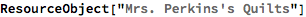Out=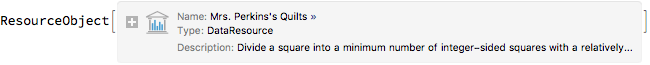Retrieve the default content:

 In:=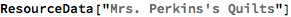Out=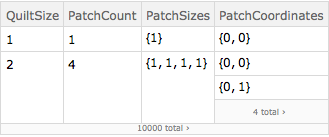Show data for the quilt of size 276:

 In:=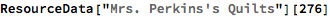Out=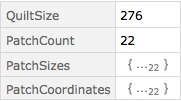Show the quilt of size 276:

 In:=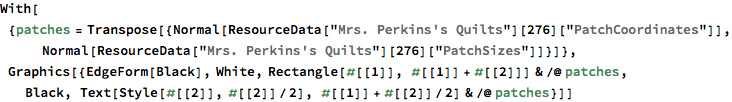Out=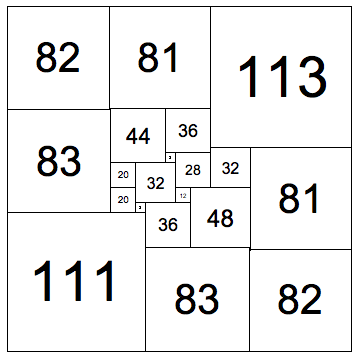Show behavior of the log(size)/order up to size 10000:

 In:=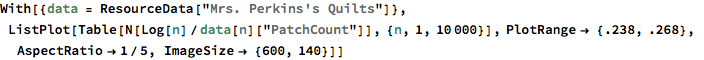Out=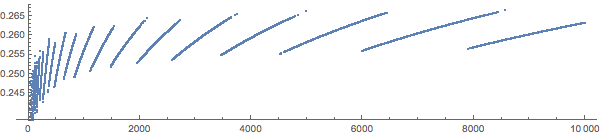Wolfram Research, "Mrs. Perkins's Quilts" from the Wolfram Data Repository (2017) https://doi.org/10.24097/wolfram.95323.data